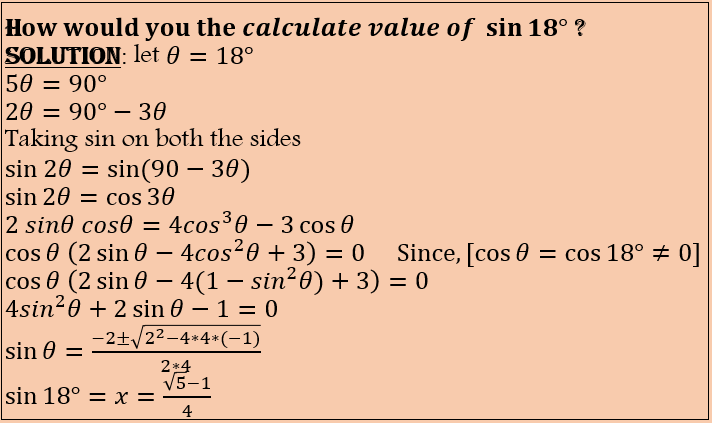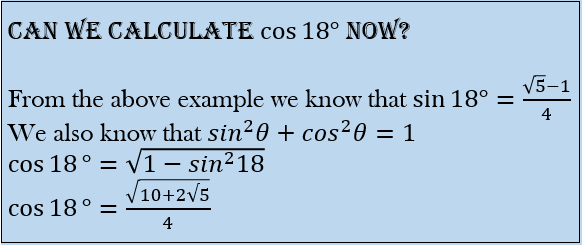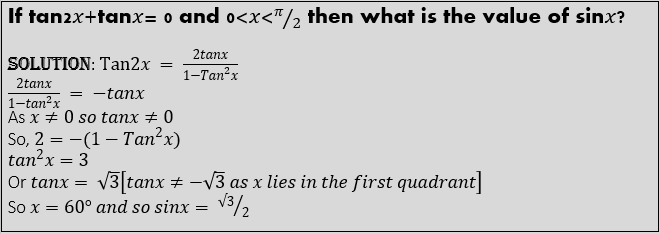# Trigonometry

#### Heights And Distances - 1

Trigonometry-1

Trigonometry

The word Trigonometry is originated from the Greek word ‘Tri’ which means three, ‘gonia’ means angle and metron means measure, i.e. it is the study of geometrical figures which have three angles. Trigonometry helps us find angles and distances. It is all about triangles…..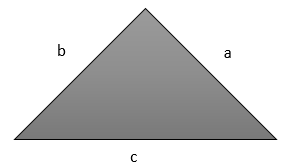The most interesting triangle is RIGHT ANGLED TRIANGLE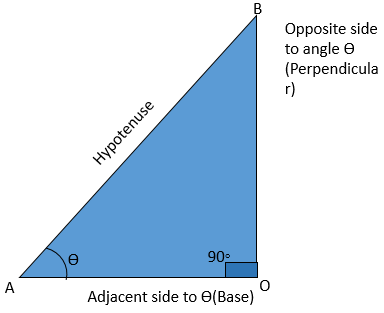We can define 6 possible ratios among the three sides of the triangle AOB, known as trigonometric ratios, they are defined as follows:Pythagorean triplets

Pythagorean triplets consists of 3 positive numbers a, b, c which satisfies Pythagoras theorem a2=b2+c2

Some examples are as follows:

(3, 4, 5)

(6, 8, 10)

(5, 12, 13)

(7, 24, 25)

(8, 15, 17)

(9, 40, 41)

The Pythagorean triplets describe the 3 integer side lengths of right angle triangle. However, right triangles with non-integer sides do not form Pythagorean triples. E.g., a = b = 1 so c =√2, But (1, 1,√2) is not a Pythagorean triplet.

Trigonometric angles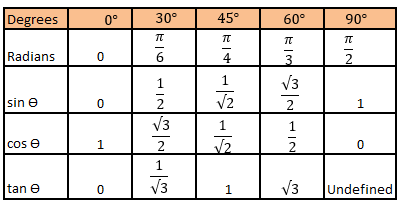Learn how to calculate base and perpendicular of a right angled triangle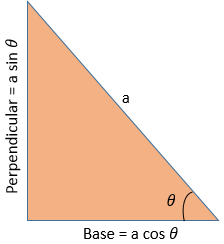Pythagorean identities

1) sin2θ+ cos2θ=1

2) 1+tan2θ= sec2θ

3) 1+ cot2θ=cosec2θ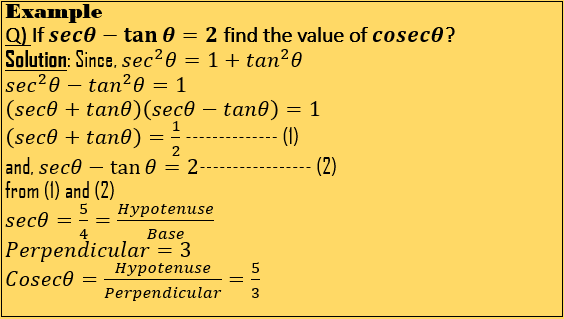Odd Even identities

sin (-θ) = -sinθ

cos (-θ) = cosθ

tan (-θ) = -tanθ

cot (-θ) = -cotθ

(-θ) = -cosecθ

sec (-θ) = secθ

ASTC rule aka also Sin Tan Cos rule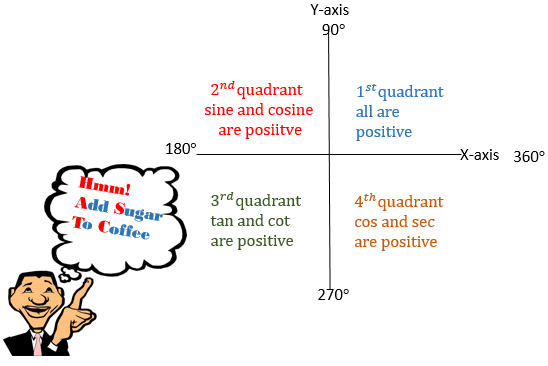* NOTE: The ASTC rule is to be read in clock wise direction, this means (90 +) will lie in 2nd quadrant

‘Sine’ will change to ‘cos’ and ‘tan’ will change to ‘cot’ and ‘cos’ will change to ‘sec’ and vice versa when angle is in the form 90K±θ where k is odd, otherwise there will be no changes.

1. When lies in the first quadrant then all trigonometric ratios are positive.
2) When  lies in the second quadrant then sine and cosecant are positive other trigonometric ratios sin, cosec, tan and cot are negative.
3) When  lies in the third quadrant then tangent and cotangent are positive other trigonometric ratios sin, cosec, tan and cot are negative.
4) When  lies in the fourth quadrant then cosine and secant are positive other trigonometric ratios sin, cosec, tan and cot are negative.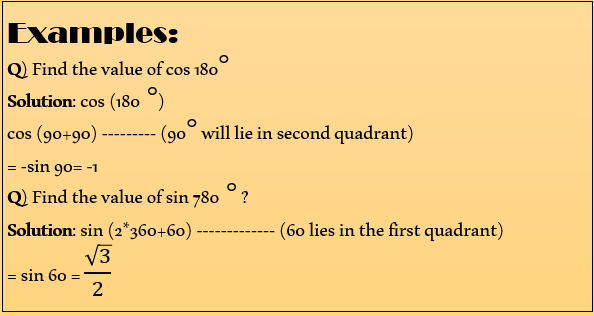Other formulae

sin a+b =sin a cos b +cos asin b

sin a-b =sinacos b-cos asin b

cos (a+b) =cos acos b-sin asin b

cos a-b =cos acos b+sin asin b